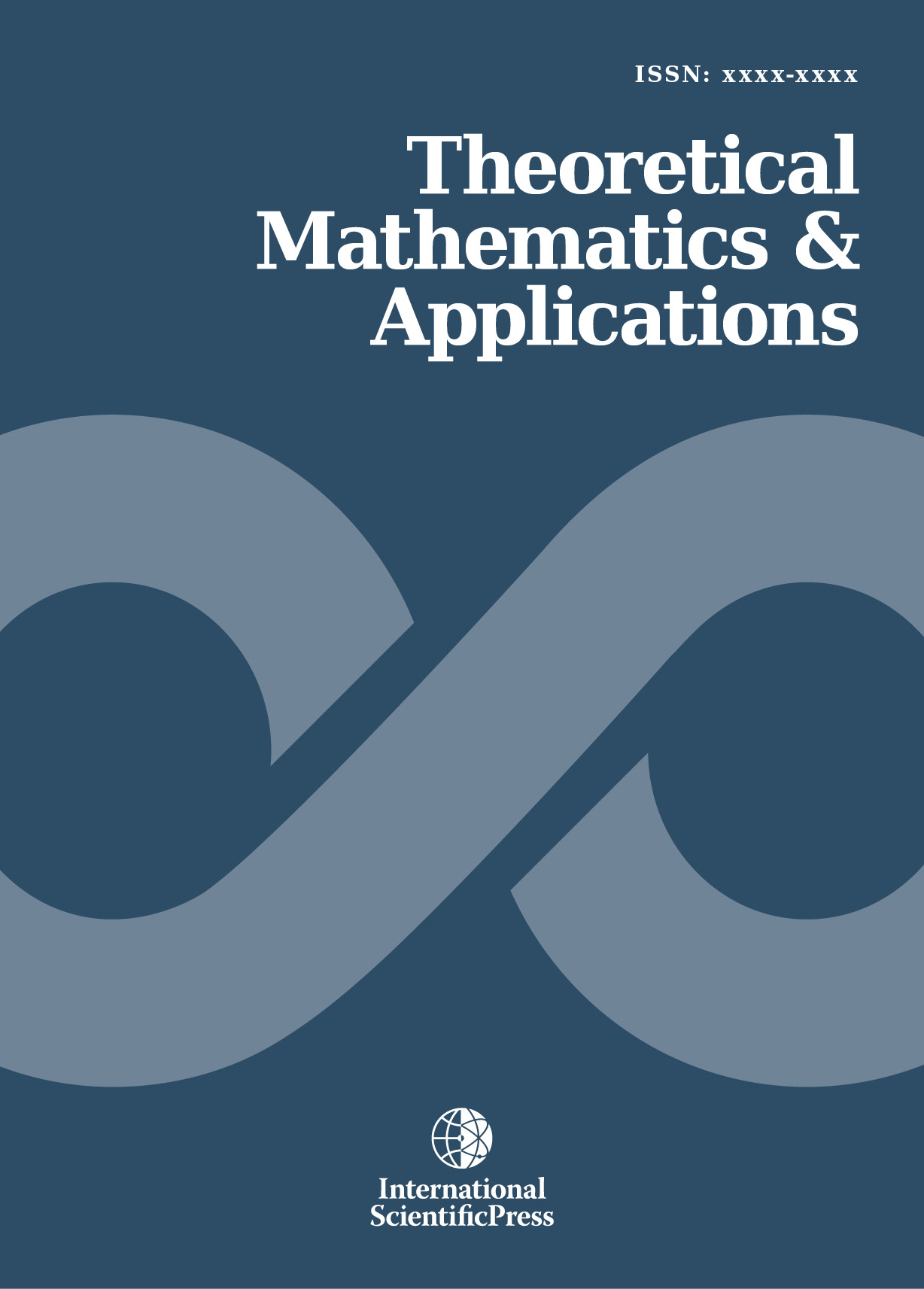# Theoretical Mathematics & Applications

#### Three remarkable properties of the Normal distribution for sample variance

•[ Download ]
• Times downloaded: 1316
• Abstract

In this paper, we present three remarkable properties of the normal distribution: first that if two independent variables ’s sum is normally distributed, then each random variable follows a normal distribution (which is referred to as the Levy Cramer theorem), second a variation of the Levy Cramer theorem (new to our knowledge) that states that two independent symmetric random variables with finite variance, independent sum and difference are necessarily normal, and third that normal distribution can be characterized by the fact that it is the only distribution for which sample mean and variance are independent, which is a central property for deriving the Student distribution and referred as the Geary theorem. The novelty of this paper is twofold. First we provide an extension of the Levy Cramer theorem. Second, for the two seminal theorem (the Levy Cramer and Geary theorem), we provide new, quicker or self contained proofs.

Mathematics Subject Classification: 62E10; 62E15
Keywords: Geary theorem; Levy Cramer theorem; independence between sample mean and varianceISSN: 1792-9687 (Print)
1792-9709 (Online)